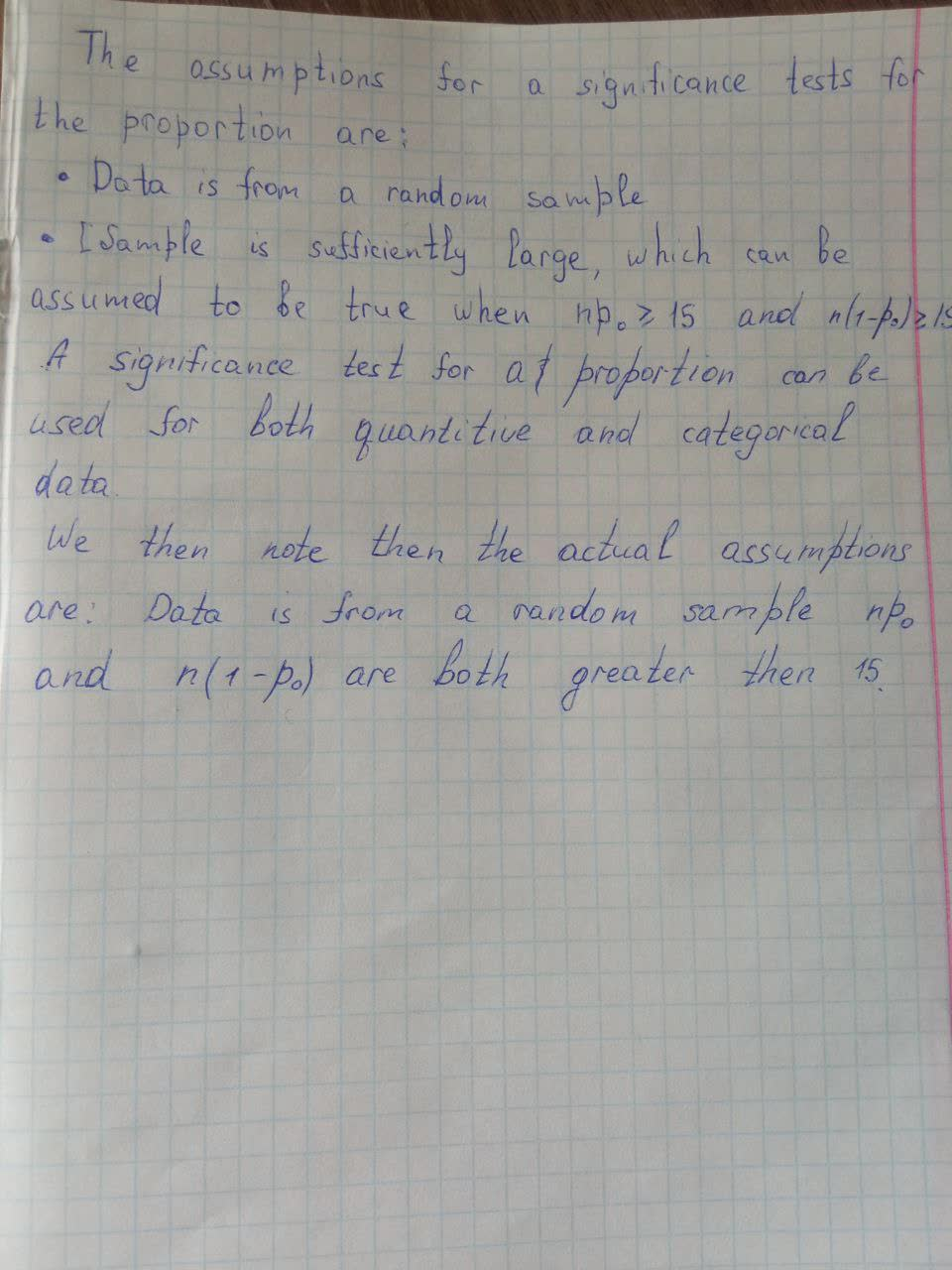Question# Find a nonzero vector orthogonal to the plane through the points P, Q, and R, P(0,-2,0), Q(4,1,-2), R(5,3,1)

Vectors
ANSWEREDFind a nonzero vector orthogonal to the plane through the points P, Q, and R, P(0,-2,0), Q(4,1,-2), R(5,3,1)2021-05-042021-09-07

The assumptions for a signicance tests for the proportion are:

- Data is from a random sample

- Sample is sufficiently large, which can be assumed to be true when $$np_o\geq15$$ and $$n(1-p_0)\geq15$$

A significane test for a proportion can be used for both quantitive and categorical data

We then note the the actual assumptions are: Data is from a random sample $$np_0$$ and $$n(1-p_0)$$ are both greater then 15.